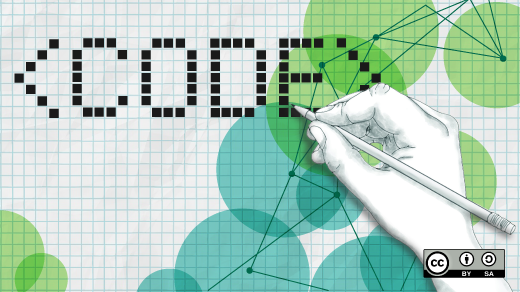Learn how to classify images with TensorFlow
• 1,801 views,
• 2018-08-02,
• 上傳者: Kuann Hung,
•  01.

```curl -O http://download.tensorflow.org/example_images/flower_photos.tgz
```
2.

```curl -O https://raw.githubusercontent.com/tensorflow/tensorflow/10cf65b48e1b2f16eaa826d2793cb67207a085d0/tensorflow/examples/image_retraining/retrain.py
```
3.

```python retrain.py --image_dir flower_photos --output_graph output_graph.pb --output_labels output_labels.txt
```
4.

```import tensorflow as tf, sys

image_path = sys.argv
graph_path = 'output_graph.pb'
labels_path = 'output_labels.txt'

# Loads label file, strips off carriage return
label_lines = [line.rstrip() for line
in tf.gfile.GFile(labels_path)]

# Unpersists graph from file
with tf.gfile.FastGFile(graph_path, 'rb') as f:
graph_def = tf.GraphDef()
_ = tf.import_graph_def(graph_def, name='')

# Feed the image_data as input to the graph and get first prediction
with tf.Session() as sess:
softmax_tensor = sess.graph.get_tensor_by_name('final_result:0')
predictions = sess.run(softmax_tensor,
{'DecodeJpeg/contents:0': image_data})
# Sort to show labels of first prediction in order of confidence
top_k = predictions.argsort()[-len(predictions):][::-1]
for node_id in top_k:
human_string = label_lines[node_id]
score = predictions[node_id]
print('%s (score = %.5f)' % (human_string, score))```
5.

```tensorboard --logdir /tmp/retrain_logs
```

資料夾 :
發表時間 :
2018-08-02 21:33:11
觀看數 :
1,801
發表人 :
Kuann Hung
部門 :
老洪的 IT 學習系統
QR Code :